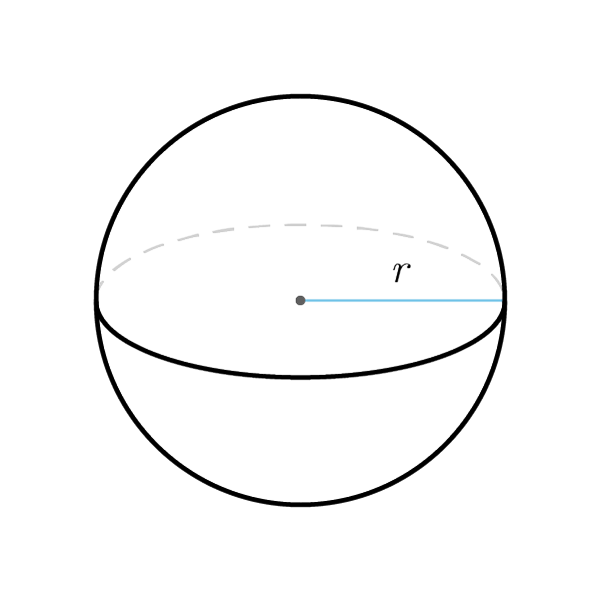# Sphere

 Radius $$r$$ Diameter $$d$$ Circumference $$C$$
$$V = \frac{4}{3} \pi {r}^{3}$$
Volume
$$r = \sqrt{\frac{3 V}{4 \pi}}$$
$$S_{tot} = 4 \pi {r}^{2}$$
Total surface
$$r = \sqrt{\frac{S_{tot}}{4 \pi}}$$
$$A = \pi {r}^{2}$$
Area
$$r = \sqrt{\frac{A}{\pi}}$$
$$C = 2 \pi r$$
Circumference
$$r = \frac{C}{2\pi}$$
$$d = 2r$$
Diameter
$$r = \frac{d}{2}$$

## Definition

A sphere is a solid made up from all points with a distance equal or less than a costant distance, called radius of the sphere, from a fixed point, called center of the sphere.

## Properties

1. The sphere is a solid generated from the rotation of a semicircle around its diameter
2. Radius: any segment that extends from the center of the sphere to a any point of the spherical surface
3. Pi (symbol $\pi$) constant value approximated as $$\pi \simeq 3.14$$
4. All the  Circle formulas are validSphere Formulas
Data Formula
Volume V = 4/3 × π × r3
Radius V = 3√[(3V) / (4π)]
Total surface Stot = 4 × π × r2
Radius r = √[Slat / (4π)]
Area A = πr2
Radius r = √(A / π)
Circumference C = 2πr
Radius r = C / (2π)
Diameter d = 2r